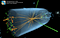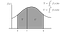# Two Myths of Numerical Integration, Debunked

Many programmers believe that the use of higher order integration algorithms, combined with a large number of integration interval divisions, is useful (and sometimes necessary) to achieve good accuracy. In this article we show that this is not always true.

# Myth No. 1: Higher Order Methods Are Better

Browsing Cantor’s Paradise articles, one of my favourite publications on Medium, I found an article written by Kazi Abu Rousan, in which the use of the trapezoidal rule for numerical integration is advocated (I am attracted by articles about physics, numerical methods and programming, as well as about the interplay between art and science). It is a well written article, in fact (as usual, on this publication) and I appreciate very much the animated figures that clearly illustrate the method. …

# Understanding the Higgs Mechanism

## A non-metaphoric explanation

If you have an elementary knowledge of physics, it is very possible that you are unsatisfied about the metaphoric explanations of the role of the Higgs boson in “giving mass to other particles”. On the other hand we do not explain gravity and electromagnetism in a similar way. We teach them using mathematical equations, whose meaning is very clear and precise, and allow for the computation of measurable quantities.An event collected by the CMS collaboration at LHC showing a Higgs boson decaying into a pair of photons (the two long green towers, representing energy deposits into the photon detector)

In a recent post by Ethan Siegel, he correctly pointed it out that most of the mass of the Universe comes, in fact, from strong interactions inside nucleons (protons and neutrons), i.e., …

# Numerical integration: the wrong way

## How a small error may lead to a disaster

Numerical integration methods are those that allow the calculation of the definite integrals of a function, using computers. Simply stated, numerical integration is the process that leads to the evaluation, in many cases approximate, of the area between a curve y=f(x) and the x-axis, in a region x∈[a,b].The integral between a and c of the function f(x) represents the grey-shaded area T; the integral between c and b is the area indicated with U. The integral between a and b represents the sum of the areas T+U. Image taken from Wikimedia Commons (thanks to Juliusross~commonswiki).

One of the simplest and, at the same time, most widely used method of numerical integration dates back to the time of Isaac Newton (this demonstrates that numerical calculation is not at all, as is believed, closely related to the use of computers). It consists of dividing the interval [a,b] into a series of N smaller intervals [xᵢ,xᵢ₊₁], approximate f(x) in each of them with a constant f(x)≃f(xᵢ)=const, and approximate the area under the curve as the sum of the areas of rectangles whose base is xᵢ₊₁-xᵢ and whose height is f(x̅), where is the midpoint of the sub-interval [xᵢ,xᵢ₊₁]. …

# 11+3 tips to solve physics problems

## A how-to guide

Practically all the exercise books I have (publishers send me some from time to time) are all the same: only the set of exercises changes. The same exercise, in fact, can be dressed in a different way and, therefore, they are not so different either. I must confess that I do not like any of them.

Rather then teaching how to solve a problem, the chapters of these books usually open with a reference to the fundamental formulas concerning the subject dealt with, and then propose a series of exercises, of increasing complexity, followed by their solution, which are almost always presented in a very clean, plain, natural and obvious form. …

# The Fermi-Dirac distribution

## Enrico Fermi became famous, in the community of physicists of the time, for having published, in 1926, an article in which he calculated the energy distribution of identical particles with half integer spin. In the same year, but a little later, Paul Dirac independently came to the same result and for this reason the distribution is now called Fermi-Dirac. Here we illustrate its meaning.

Matter, as we know, is made up of electrons, neutrons and protons, which are are particles with half-integer spin, that is particles with an intrinsic angular momentum equal to half of an integer number (in this case equal to 1/2).

The intrinsic angular momentum (or spin) is often described as a classical intrinsic angular momentum, i.e. as a quantity that measures the speed of rotation of a particle around its axis. This picture is completely wrong, in fact. The spin has no classical counterpart and certainly cannot be thought of as a classical angular momentum: particles are point-like and do not rotate around any axis. It is better to think of it as a quantity that can be measured for these particles. They can then be labeled with the values +1/2 or −1/2, which determines, along with other quantities, their state. …

# Is 10 million times 7 equal to 70 million?

## Numerical computation can lead to subtle rounding errors. Good programmers must know how to avoid them.

Consider the following program, written in C:

`#include <stdio.h>int main() { float x = 7; float S = 0; int i; for (i = 0; i < 10000000; i++) {   S += x; } printf(“%f\n”, S);}`

The program adds 7 to the variable S ten million times. The expected result is thus 70 million. Indeed, compiling (with no optimisation) and running the program gives

`77603248.000000`

That is more than 10% larger. Surprised? Well, this is the effect of rounding errors caused by the fact that numbers, in computers, can only be represented with a finite number of digits. In fact, both 7 and 10 million are perfectly representable in a computer’s memory. …

# Programming Etiquette

## Writing software is not a purely technical activity, just as writing an article for a newspaper is not a mere list of facts. It is also an exercise in style, quite similar to what you do when writing a novel.

Programming languages are always very rigid in the respect of syntactic rules (much stricter than a proofreader), but they often leave the programmer with certain freedoms that, if badly used, like all freedoms, prove to be harmful and, almost always, backfire on the author.

Through the “style” choices the programmer can convey additional messages to the content of his work. By choosing one form rather than another, to a certain extent, he/she communicate information that, often, helps the reader (often the author him/herself) to decipher the content of a program.

Variable names
The choice of names to be assigned to variables is of great importance when writing a program. Each variable must always be given a meaningful name, which recalls its meaning and how it is used in the program. The type of data contained in the variables can also be suggested by the name, which, however, must never be too long. …

# The power of beauty

## Why physical laws appear to be so beautiful

Ok, I admit it. Not every physics law looks nice and many readers will not agree with the statement above, but a large part of physics and math lovers (those that follow me) will do. So, let me comment about that.

Many scientists believe in what I call the “beauty argument”, that lead Prof. Feynman to tell that

The “beauty argument” consists in believing that Nature must be described by beautiful looking equations. I guess that such a belief stems from an interview given by Paul Dirac to Thomas Kuhn and Eugene Wigner in which one of the fathers of quantum mechanics…

# How to correctly write Newton’s second Law

Why F=ma isn’t correct.

As a matter of fact, most textbooks report the Newton’s second Law as F=ma (bold letters represent vectors). In this post I argue that this is not the correct way to write it.

Most students perceive physics as a discipline very close to mathematics. Most textbooks, indeed, teach physics the same way in which they teach mathematics: by the enunciation of sort of axioms (physics laws), followed by a set of theorem demonstrations and mathematical manipulations. Physics laws appear as axioms because they are often not justified by any experimental evidence. For most of them it is said that “it can be shown that…” or that “Newton observed that…” or even “Faraday discovered that…”. …

# Towards a demystification of quantum mechanics

## episode 5: the collapse of the wavefunction

The wavefunction was introduced in the early times of quantum mechanics as a solution of the Schrödinger equation. Physicists struggled a bit to find its meaning, and finally agreed to interpret it as the probability amplitude to find a particle at a given coordinate.

The problems arise when it collapse: according to quantum mechanics, a system can be in a superposition of states, i.e., its wavefunction can be the sum of more than one physically observable ones. It turns out that, each time we measure the state of such a system, only one of the states in the superposition manifests, while the other just disappears. …